To build these slides:

In :
%%bash
jupyter nbconvert \
--to=slides \
--reveal-prefix=https://cdnjs.cloudflare.com/ajax/libs/reveal.js/3.2.0/ \
--output=dataphilly-jul2016 \
./DataPhilly\ July\ 2016\ Introduction\ to\ Probabilistic\ Programming.ipynb

[NbConvertApp] Converting notebook ./DataPhilly July 2016 Introduction to Probabilistic Programming.ipynb to slides
[NbConvertApp] Writing 561178 bytes to ./dataphilly-jul2016.slides.html

In :
%matplotlib inline

In :
from __future__ import division

In :
from IPython.display import Image

In :
from matplotlib import pyplot as plt
import numpy as np
import pandas as pd
import scipy as sp
import seaborn as sns
from theano import tensor as T

In :
blue, green, red, purple, gold, teal = sns.color_palette()

In :
plt.rc('figure', figsize=(8, 6))

LABELSIZE = 14
plt.rc('axes', labelsize=LABELSIZE)
plt.rc('legend', fontsize=LABELSIZE)
plt.rc('xtick', labelsize=LABELSIZE)
plt.rc('ytick', labelsize=LABELSIZE)

In :
SEED = 2285280 # from random.org


# Introduction to Probabilistic Programming¶

## Probabilistic Programming¶

• Programming with random variables
• User specifies a generative model for the observed data
• "Tell the story" of how the data were created
• The language runtime/software library automatically performs inference### What do we mean by inference?¶

• Maximum likelihood inference
• Maxumum a posteriori inference
• Full posterior inference## Disease Testing¶

A certain rare disease affects one in 10,000 people. A test for the disease gives the correct result 99.9% of the time.

What is the probability that a given test is positive?

In :
import pymc3 as pm

with pm.Model() as pretest_disease_model:
# This is our prior belief about whether or not the subject has the disease,
# given its prevalence of the disease in the general population
has_disease = pm.Bernoulli('has_disease', 1e-4)

# This is the probability of a positive test given the presence or lack
# of the disease
p_positive = pm.Deterministic('p_positive',
T.switch(T.eq(has_disease, 1),
# If the subject has the disease,
# this is the probability that the
# test is positive
0.999,
# If the subject does not have the
# disease, this is the probability
# that the test is negative
1 - 0.999))

# This is the observed test result
test_positive = pm.Bernoulli('test_positive', p_positive)

In :
samples = 40000

In :
with pretest_disease_model:
step = pm.Metropolis()
pretest_disease_trace = pm.sample(samples, step, random_seed=SEED)

 [-----------------100%-----------------] 40000 of 40000 complete in 6.1 sec
In :
pretest_disease_trace['test_positive']

Out:
array([0, 0, 0, ..., 0, 0, 0])
In :
pretest_disease_trace['test_positive'].size

Out:
40000

The probability that a given test is positive is

In :
pretest_disease_trace['test_positive'].mean()

Out:
0.001575
\begin{align*} P(\textrm{Test } +) = &\ P(\textrm{Test } +\ |\ \textrm{Disease}) P(\textrm{Disease}) \\ & + P(\textrm{Test } +\ |\ \textrm{No disease}) P(\textrm{No disease}) \end{align*}
In :
0.999 * 1e-4  + (1 - 0.999) * (1 - 1e-4)

Out:
0.0010998000000000008

If a subject tests positive, what is the probability they have the disease?

In :
with pm.Model() as disease_model:
# This is our prior belief about whether or not the subject has the disease,
# given its prevalence of the disease in the general population
has_disease = pm.Bernoulli('has_disease', 1e-4)

# This is the probability of a positive test given the presence or lack
# of the disease
p_positive = pm.Deterministic('p_positive',
T.switch(T.eq(has_disease, 1),
# If the subject has the disease,
# this is the probability that the
# test is positive
0.999,
# If the subject does not have the
# disease, this is the probability
# that the test is negative
1 - 0.999))

# This is the observed positive test
test_positive = pm.Bernoulli('test_positive', p_positive, observed=1)

In :
with disease_model:
step = pm.Metropolis()
disease_trace = pm.sample(samples, step, random_seed=SEED)

 [-----------------100%-----------------] 40000 of 40000 complete in 3.8 sec

The probability that someone who tests positive for the disease has it is

In :
disease_trace['has_disease'].mean()

Out:
0.096775
\begin{align*} P(\textrm{Disease}\ |\ \textrm{Test }+) & = \frac{P(\textrm{Test }+\ |\ \textrm{Disease}) P(\textrm{Disease})}{P(\textrm{Test }+)} \end{align*}
In :
0.999 * 1e-4 / (0.999 * 1e-4 + (1 - 0.999) * (1 - 1e-4))

Out:
0.09083469721767587

## The Monty Hall Problem¶If we select the first door and Monty opens the third to reveal a goat, should we change doors?

In :
with pm.Model() as monty_model:
# We have no idea where the prize is at the beginning
prize = pm.DiscreteUniform('prize', 0, 2)

# The probability that Monty opens each door
p_open = pm.Deterministic('p_open',
T.switch(T.eq(prize, 0),
# If the prize is behind the first door,
# he chooses to open one of the others
# at random
np.array([0., 0.5, 0.5]),
T.switch(T.eq(prize, 1),
# If it is behind the second door,
# he must open the third door
np.array([0., 0., 1.]),
# If it is behind the third door,
# he must open the second door
np.array([0., 1., 0.]))))

# Monty opened the third door, revealing a goat
opened = pm.Categorical('opened', p_open, observed=2)

In :
samples = 10000

In :
with monty_model:
step = pm.Metropolis()
monty_trace = pm.sample(samples, step, random_seed=SEED)

monty_trace_df = pm.trace_to_dataframe(monty_trace)

 [-----------------100%-----------------] 10000 of 10000 complete in 1.4 sec
In :
monty_trace_df.head()

Out:
p_open__0 p_open__1 p_open__2 prize
0 0.0 0.0 1.0 1
1 0.0 0.5 0.5 0
2 0.0 0.0 1.0 1
3 0.0 0.0 1.0 1
4 0.0 0.0 1.0 1
In :
(monty_trace_df.groupby('prize')
.size()
.div(monty_trace_df.shape))

Out:
prize
0    0.3196
1    0.6804
dtype: float64

## PyMC3¶

PyMC3 is a python module for Bayesian statistical modeling and model fitting which focuses on advanced Markov chain Monte Carlo fitting algorithms. Its flexibility and extensibility make it applicable to a large suite of problems.

Built on top of theano## A/B Testing¶Suppose variant A sees 510 visitors and 40 purchases, but variant B sees 505 visitors and 50 conversions.

In :
with pm.Model() as ab_model:
# A priori, we have no idea what the purchase rate for variant A will be
a_rate = pm.Uniform('a_rate')
# We observed 40 purchases from 510 visitors who saw variant A
a_purchases = pm.Binomial('a_purchases', 510, a_rate, observed=40)

# A priori, we have no idea what the purchase rate for variant B will be
b_rate = pm.Uniform('b_rate')
# We observed 40 purchases from 510 visitors who saw variant A
b_purchases = pm.Binomial('b_purchases', 505, b_rate, observed=45)

Applied interval-transform to a_rate and added transformed a_rate_interval to model.
Applied interval-transform to b_rate and added transformed b_rate_interval to model.

In :
samples = 10000

In :
with ab_model:
step = pm.NUTS()
ab_trace = pm.sample(samples, step, random_seed=SEED)

 [-----------------100%-----------------] 10000 of 10000 complete in 3.2 sec
In :
fig, ax = plt.subplots(figsize=(8, 6))

BINS = 30

ax.hist(np.clip(ab_trace['a_rate'], 0.03, 0.15),
bins=BINS, normed=True, lw=0, alpha=0.75,
label="Variant A");
ax.hist(np.clip(ab_trace['b_rate'], 0.03, 0.15),
bins=BINS, normed=True, lw=0, alpha=0.75,
label="Variant B");

ax.set_xlabel('Purchase rate');
ax.set_yticklabels([]);
ax.legend();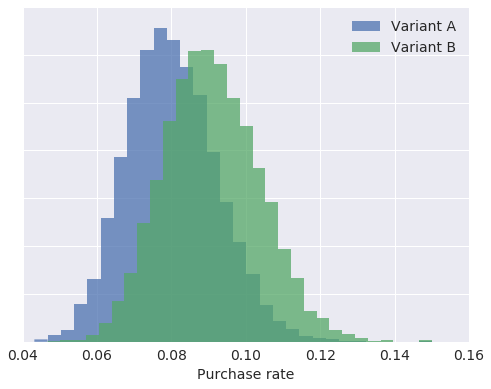In :
fig

Out:The probability that variant B is better than variant A is

In :
(ab_trace['a_rate'] < ab_trace['b_rate']).mean()

Out:
0.7218

## Robust Regression¶

In :
x = np.array([10, 8, 13, 9, 11, 14, 6, 4, 12, 7, 5])
y = np.array([7.46, 6.77, 12.74, 7.11, 7.81, 8.84, 6.08, 5.39, 8.15, 6.42, 5.73])

In :
fig, ax = plt.subplots(figsize=(8, 6))

ax.scatter(x, y, c='k', s=50);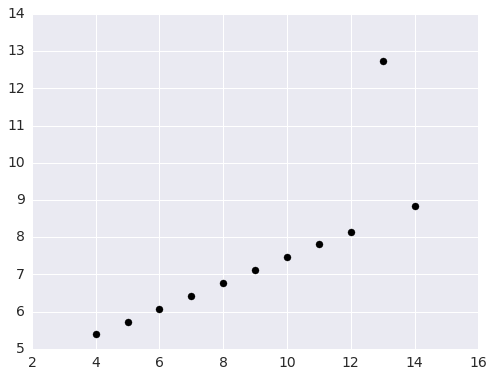In :
fig

Out:In :
X = np.empty((x.size, 2))
X[:, 0] = 1
X[:, 1] = x

coef, _, __, ___ = np.linalg.lstsq(X, y)

In :
plot_X = np.ones((100, 2))

x_min, x_max = x.min() - 1, x.max() + 1
plot_X[:, 1] = np.linspace(x_min, x_max, 100)

In :
fig, ax = plt.subplots(figsize=(8, 6))

ax.scatter(x, y, c='k', s=50);
ax.plot(plot_X[:, 1], plot_X.dot(coef),
c='k', label='Least squares');

ax.set_xlim(x_min, x_max);
ax.legend(loc=2);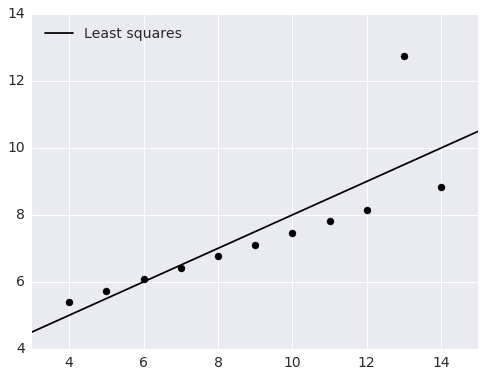In :
fig

Out:In :
with pm.Model() as ols_model:
# alpha is the slope and beta is the intercept
alpha = pm.Uniform('alpha', -100, 100)
beta = pm.Uniform('beta', -100, 100)

# Ordinary least squares assumes that the noise is normally distributed
y_obs = pm.Normal('y_obs', alpha + beta * x, 1., observed=y)

Applied interval-transform to alpha and added transformed alpha_interval to model.
Applied interval-transform to beta and added transformed beta_interval to model.

In :
samples = 10000

In :
with ols_model:
step = pm.Metropolis()
ols_trace = pm.sample(samples, step, random_seed=SEED)

 [-----------------100%-----------------] 10000 of 10000 complete in 2.2 sec
In :
post_y = ols_trace['alpha'] + np.outer(plot_X[:, 1], ols_trace['beta'])

In :
fig, ax = plt.subplots(figsize=(8, 6))

ax.fill_between(plot_X[:, 1],
np.percentile(post_y, 2.5, axis=1),
np.percentile(post_y, 97.5, axis=1),
color='gray', alpha=0.5,
label='95% CI');
ax.scatter(x, y, c='k', s=50);
ax.plot(plot_X[:, 1], plot_X.dot(coef),
c='k', label='Least squares');
ax.plot(plot_X[:, 1], post_y.mean(axis=1),
c='r', ls='--', label='PyMC3 least squares');

ax.set_xlim(x_min, x_max);
ax.legend(loc=2);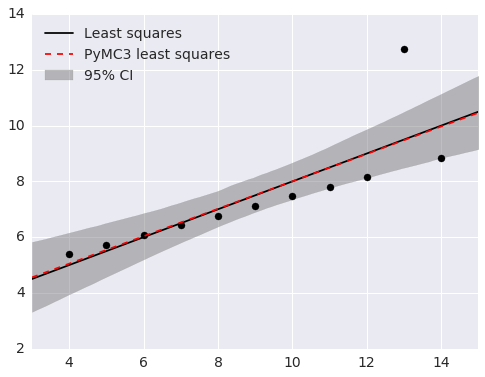In :
fig

Out:In :
fig, ax = plt.subplots(figsize=(8, 6))

z = np.linspace(-4, 4, 100)

ax.plot(z, sp.stats.norm.pdf(z),
label='$N(0, 1)$');
ax.plot(z, sp.stats.t.pdf(z, 3),
label=r'$t(\nu = 3)$');

ax.set_xlabel('Residual');
ax.set_yticklabels([]);
ax.set_ylabel('Probability density');
ax.legend();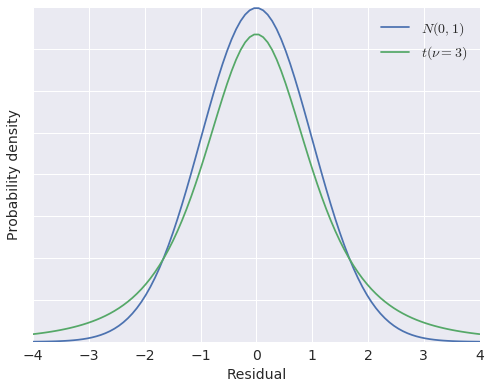In :
fig

Out:In :
with pm.Model() as robust_model:
# alpha is the slope and beta is the intercept
alpha = pm.Uniform('alpha', -100, 100)
beta = pm.Uniform('beta', -100, 100)

# t-distributed residuals are less sensitive to outliers
y_obs = pm.StudentT('y_obs', nu=3., mu=alpha + beta * x, observed=y)

Applied interval-transform to alpha and added transformed alpha_interval to model.
Applied interval-transform to beta and added transformed beta_interval to model.

In :
with robust_model:
step = pm.Metropolis()

robust_trace = pm.sample(samples, step, random_seed=SEED)

 [-----------------100%-----------------] 10000 of 10000 complete in 2.3 sec
In :
post_y_robust = robust_trace['alpha'] + np.outer(plot_X[:, 1], robust_trace['beta'])

In :
fig, (l_ax, r_ax) = plt.subplots(ncols=2, sharex=True, sharey=True, figsize=(16, 6))

l_ax.fill_between(plot_X[:, 1],
np.percentile(post_y, 2.5, axis=1),
np.percentile(post_y, 97.5, axis=1),
color='gray', alpha=0.5,
label='95% CI');
l_ax.scatter(x, y, c='k', s=50);
l_ax.plot(plot_X[:, 1], plot_X.dot(coef),
c='k', label='Least squares');
l_ax.plot(plot_X[:, 1], post_y.mean(axis=1),
c='r', ls='--', label='PyMC3 least squares');

l_ax.set_xlim(x_min, x_max);
l_ax.legend(loc=2);

r_ax.fill_between(plot_X[:, 1],
np.percentile(post_y_robust, 2.5, axis=1),
np.percentile(post_y_robust, 97.5, axis=1),
color='gray', alpha=0.5,
label='95% CI');
r_ax.scatter(x, y, c='k', s=50);
r_ax.plot(plot_X[:, 1], post_y_robust.mean(axis=1),
c=blue, label='PyMC3 robust');

r_ax.set_xlim(x_min, x_max);
r_ax.legend(loc=2);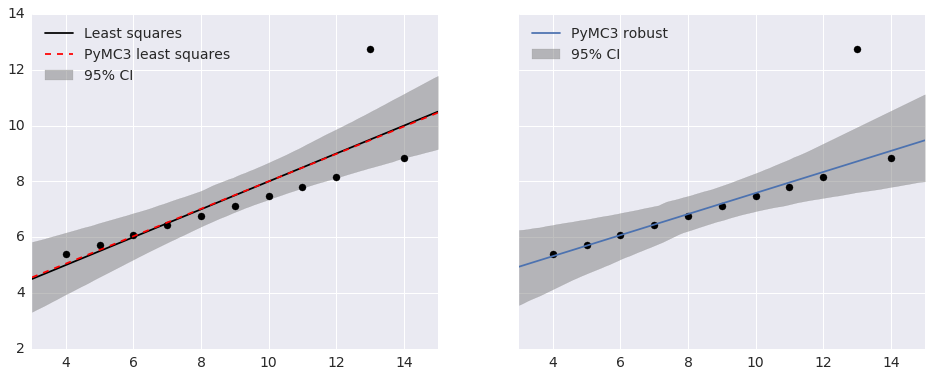In :
fig

Out:## Congressional Ideal Point Model¶

In :
vote_data_uri = 'http://archive.ics.uci.edu/ml/machine-learning-databases/voting-records/house-votes-84.data'

In :
N_BILLS = 16
BILLS = list(range(N_BILLS))

df = pd.read_csv(vote_data_uri, names=['party'] + BILLS)

df.index.name = 'rep'

df[df == 'n'] = 0
df[df == 'y'] = 1
df[df == '?'] = np.nan

df.party, parties = df.party.factorize()

N_REPS = df.shape

In :
df.head()

Out:
party 0 1 2 3 4 5 6 7 8 9 10 11 12 13 14 15
rep
0 0 0 1 0 1 1 1 0 0 0 1 NaN 1 1 1 0 1
1 0 0 1 0 1 1 1 0 0 0 0 0 1 1 1 0 NaN
2 1 NaN 1 1 NaN 1 1 0 0 0 0 1 0 1 1 0 0
3 1 0 1 1 0 NaN 1 0 0 0 0 1 0 1 0 0 1
4 1 1 1 1 0 1 1 0 0 0 0 1 NaN 1 1 1 1
In :
parties

Out:
Index(['republican', 'democrat'], dtype='object')
In :
vote_df = (pd.melt(df.reset_index(),
id_vars=['rep', 'party'], value_vars=BILLS,
var_name='bill', value_name='vote')
.dropna()
.astype(np.int64))

In :
grid = sns.factorplot('bill', 'vote', 'party', vote_df,
kind='bar', ci=None, size=8, aspect=1.5);
grid.set_axis_labels('Bill', 'Percent voting for bill');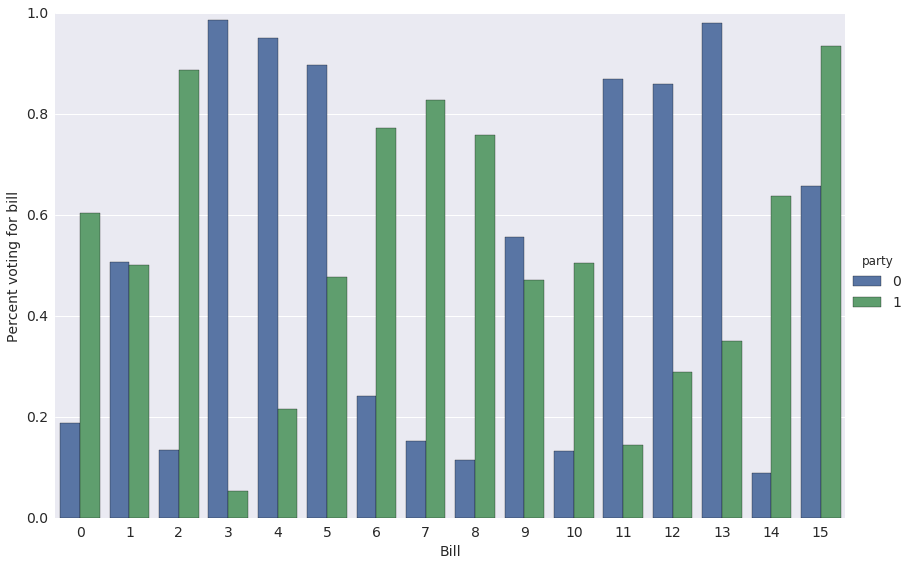In :
grid.fig

Out:$p_{i, j}$ is the probability that representative $i$ votes for bill $j$.

\begin{align*} \log \left(\frac{p_{i, j}}{1 - p_{i, j}}\right) & = \gamma_j \left(\alpha_i - \beta_j\right) \\ & = \textrm{ability of bill } j \textrm{ to discriminate} \times (\textrm{conservativity of represetative } i\ - \textrm{conservativity of bill } j) \end{align*}
In :
with pm.Model() as ideal_point_model:
# The conservativity of representative i
alpha = pm.Normal('alpha', 0, 1., shape=N_REPS)

# The conservativity of bill j
mu_beta = pm.Normal('mu_beta', 0, 1e-3)
sigma_beta = pm.Uniform('sigma_beta', 0, 1e2)
beta = pm.Normal('beta', mu_beta, sd=sigma_beta, shape=N_BILLS)

# The ability of bill j to discriminate
mu_gamma = pm.Normal('mu_gamma', 0, 1e-3)
sigma_gamma = pm.Uniform('sigma_gamma', 0, 1e2)
gamma = pm.Normal('gamma', mu_gamma, sd=sigma_gamma, shape=N_BILLS)

# The probability that representative i votes for bill j
p = pm.Deterministic('p', T.nnet.sigmoid(gamma[vote_df.bill] * (alpha[vote_df.rep] - beta[vote_df.bill])))

vote = pm.Bernoulli('vote', p, observed=vote_df.vote)

Applied interval-transform to sigma_beta and added transformed sigma_beta_interval to model.
Applied interval-transform to sigma_gamma and added transformed sigma_gamma_interval to model.

In :
samples = 20000

In :
with ideal_point_model:
step = pm.Metropolis()
ideal_point_trace = pm.sample(samples, step, random_seed=SEED)

 [-----------------100%-----------------] 20000 of 20000 complete in 107.5 sec
In :
rep_alpha = ideal_point_trace['alpha'].mean(axis=0)

In :
fig, ax = plt.subplots(figsize=(8, 6))

ax.hist(rep_alpha[(df.party == (parties == 'republican').argmax()).values],
normed=True, bins=10, color=red, alpha=0.75, lw=0,
label='Republicans');
ax.hist(rep_alpha[(df.party == (parties == 'democrat').argmax()).values],
normed=True, bins=10, color=blue, alpha=0.75, lw=0,
label='Democrats');

ax.set_xlabel(r'$\alpha$');
ax.set_yticklabels([]);
ax.set_ylabel('Probability density');
ax.legend(loc=1);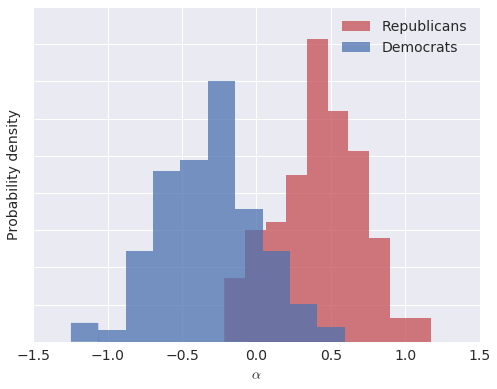In :
fig

Out:In :
pm.forestplot(ideal_point_trace, varnames=['gamma']);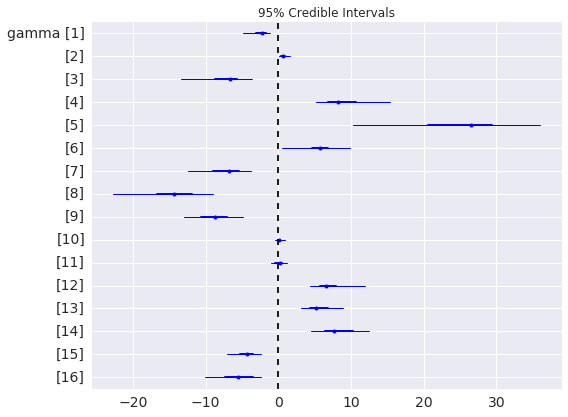## Probabilistic Programming Ecosystem¶

Probabilistic Programming System Language Discrete Variable Support Automatic Differentiation/Hamiltonian Monte Carlo Variational Inference
PyMC3 Python 2/3PyMC2 Python 2/3Stan C++, R, Python 2/3BUGS Standalone program, RJAGS Standalone program, RProbabilistic Programming and Bayesian Methods for Hackers (Open source, uses PyMC2, PyMC3 port in progress)Think Bayes (Available freely online, calculations in Python)Bayesian Data Analysis## Thank You!¶

The Jupyter notebook these slides were generated from is available here